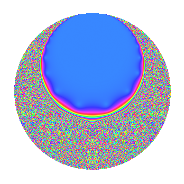Properties

 Label 8.18.a.bLevel 8 Weight 18 Character orbit 8.a Self dual Yes Analytic conductor 14.658 Analytic rank 1 Dimension 2 CM No Inner twists 1

Related objects

Newspace parameters

 Level: $$N$$ = $$8 = 2^{3}$$ Weight: $$k$$ = $$18$$ Character orbit: $$[\chi]$$ = 8.a (trivial)

Newform invariants

 Self dual: Yes Analytic conductor: $$14.6577669876$$ Analytic rank: $$1$$ Dimension: $$2$$ Coefficient field: $$\Q(\sqrt{114})$$ Coefficient ring: $$\Z[a_1, a_2, a_3]$$ Coefficient ring index: $$2^{7}\cdot 3^{2}$$ Fricke sign: $$1$$ Sato-Tate group: $\mathrm{SU}(2)$

$q$-expansion

Coefficients of the $$q$$-expansion are expressed in terms of $$\beta = 1152\sqrt{114}$$. We also show the integral $$q$$-expansion of the trace form.

 $$f(q)$$ $$=$$ $$q$$ $$+ ( 5796 + \beta ) q^{3}$$ $$+ ( -395962 - 68 \beta ) q^{5}$$ $$+ ( -9466296 - 1582 \beta ) q^{7}$$ $$+ ( 55743309 + 11592 \beta ) q^{9}$$ $$+O(q^{10})$$ $$q$$ $$+ ( 5796 + \beta ) q^{3}$$ $$+ ( -395962 - 68 \beta ) q^{5}$$ $$+ ( -9466296 - 1582 \beta ) q^{7}$$ $$+ ( 55743309 + 11592 \beta ) q^{9}$$ $$+ ( -235385620 - 10269 \beta ) q^{11}$$ $$+ ( -1251761714 + 49948 \beta ) q^{13}$$ $$+ ( -12582705960 - 790090 \beta ) q^{15}$$ $$+ ( -24222344174 + 1366664 \beta ) q^{17}$$ $$+ ( -41241650380 + 6247141 \beta ) q^{19}$$ $$+ ( -294207203808 - 18635568 \beta ) q^{21}$$ $$+ ( -153548515624 - 7579978 \beta ) q^{23}$$ $$+ ( 93410746463 + 53850832 \beta ) q^{25}$$ $$+ ( 1328343844968 - 6209622 \beta ) q^{27}$$ $$+ ( 994787693790 + 46341932 \beta ) q^{29}$$ $$+ ( 5376066787616 - 263508856 \beta ) q^{31}$$ $$+ ( -2917890584784 - 294904744 \beta ) q^{33}$$ $$+ ( 20023451045808 + 1270120012 \beta ) q^{35}$$ $$+ ( -25587226374810 - 441997556 \beta ) q^{37}$$ $$+ ( 301414833144 - 962263106 \beta ) q^{39}$$ $$+ ( -57113645522262 + 2940525904 \beta ) q^{41}$$ $$+ ( -28378777101812 - 4230485749 \beta ) q^{43}$$ $$+ ( -141327368849394 - 8380536516 \beta ) q^{45}$$ $$+ ( 100776581579184 + 10149312940 \beta ) q^{47}$$ $$+ ( 235616999540153 + 29951360544 \beta ) q^{49}$$ $$+ ( 66369692927880 - 16301159630 \beta ) q^{51}$$ $$+ ( 108637731793526 - 56505234100 \beta ) q^{53}$$ $$+ ( 198848256992392 + 20072355938 \beta ) q^{55}$$ $$+ ( 706092456699216 - 5033221144 \beta ) q^{57}$$ $$+ ( -233155724445028 + 65093222991 \beta ) q^{59}$$ $$+ ( 1031908631813374 + 101795756156 \beta ) q^{61}$$ $$+ ( -3302118344023128 - 197919218070 \beta ) q^{63}$$ $$+ ( -18200477670316 + 65342286576 \beta ) q^{65}$$ $$+ ( -3318612981179164 - 53283505807 \beta ) q^{67}$$ $$+ ( -2036740976659872 - 197482068112 \beta ) q^{69}$$ $$+ ( 771842498992264 + 360055439394 \beta ) q^{71}$$ $$+ ( -1867053400179254 - 288694867608 \beta ) q^{73}$$ $$+ ( 8688493305259740 + 405530168735 \beta ) q^{75}$$ $$+ ( 4686018083523168 + 469589444464 \beta ) q^{77}$$ $$+ ( 8877689253665488 - 575220781772 \beta ) q^{79}$$ $$+ ( -439071903179271 - 204639893640 \beta ) q^{81}$$ $$+ ( 12726798779079028 - 1526245359211 \beta ) q^{83}$$ $$+ ( -4468715339880724 + 1105972393064 \beta ) q^{85}$$ $$+ ( 12776853692248632 + 1263385531662 \beta ) q^{87}$$ $$+ ( -33608419329601830 - 1843251450264 \beta ) q^{89}$$ $$+ ( -105034994694672 + 1507464478940 \beta ) q^{91}$$ $$+ ( -8706533777942400 + 3848769458240 \beta ) q^{93}$$ $$+ ( -47938649868749768 + 330801781198 \beta ) q^{95}$$ $$+ ( -65489512430721694 - 6403182311000 \beta ) q^{97}$$ $$+ ( -31130452748228868 - 3301018147161 \beta ) q^{99}$$ $$+O(q^{100})$$ $$\operatorname{Tr}(f)(q)$$ $$=$$ $$2q$$ $$\mathstrut +\mathstrut 11592q^{3}$$ $$\mathstrut -\mathstrut 791924q^{5}$$ $$\mathstrut -\mathstrut 18932592q^{7}$$ $$\mathstrut +\mathstrut 111486618q^{9}$$ $$\mathstrut +\mathstrut O(q^{10})$$ $$2q$$ $$\mathstrut +\mathstrut 11592q^{3}$$ $$\mathstrut -\mathstrut 791924q^{5}$$ $$\mathstrut -\mathstrut 18932592q^{7}$$ $$\mathstrut +\mathstrut 111486618q^{9}$$ $$\mathstrut -\mathstrut 470771240q^{11}$$ $$\mathstrut -\mathstrut 2503523428q^{13}$$ $$\mathstrut -\mathstrut 25165411920q^{15}$$ $$\mathstrut -\mathstrut 48444688348q^{17}$$ $$\mathstrut -\mathstrut 82483300760q^{19}$$ $$\mathstrut -\mathstrut 588414407616q^{21}$$ $$\mathstrut -\mathstrut 307097031248q^{23}$$ $$\mathstrut +\mathstrut 186821492926q^{25}$$ $$\mathstrut +\mathstrut 2656687689936q^{27}$$ $$\mathstrut +\mathstrut 1989575387580q^{29}$$ $$\mathstrut +\mathstrut 10752133575232q^{31}$$ $$\mathstrut -\mathstrut 5835781169568q^{33}$$ $$\mathstrut +\mathstrut 40046902091616q^{35}$$ $$\mathstrut -\mathstrut 51174452749620q^{37}$$ $$\mathstrut +\mathstrut 602829666288q^{39}$$ $$\mathstrut -\mathstrut 114227291044524q^{41}$$ $$\mathstrut -\mathstrut 56757554203624q^{43}$$ $$\mathstrut -\mathstrut 282654737698788q^{45}$$ $$\mathstrut +\mathstrut 201553163158368q^{47}$$ $$\mathstrut +\mathstrut 471233999080306q^{49}$$ $$\mathstrut +\mathstrut 132739385855760q^{51}$$ $$\mathstrut +\mathstrut 217275463587052q^{53}$$ $$\mathstrut +\mathstrut 397696513984784q^{55}$$ $$\mathstrut +\mathstrut 1412184913398432q^{57}$$ $$\mathstrut -\mathstrut 466311448890056q^{59}$$ $$\mathstrut +\mathstrut 2063817263626748q^{61}$$ $$\mathstrut -\mathstrut 6604236688046256q^{63}$$ $$\mathstrut -\mathstrut 36400955340632q^{65}$$ $$\mathstrut -\mathstrut 6637225962358328q^{67}$$ $$\mathstrut -\mathstrut 4073481953319744q^{69}$$ $$\mathstrut +\mathstrut 1543684997984528q^{71}$$ $$\mathstrut -\mathstrut 3734106800358508q^{73}$$ $$\mathstrut +\mathstrut 17376986610519480q^{75}$$ $$\mathstrut +\mathstrut 9372036167046336q^{77}$$ $$\mathstrut +\mathstrut 17755378507330976q^{79}$$ $$\mathstrut -\mathstrut 878143806358542q^{81}$$ $$\mathstrut +\mathstrut 25453597558158056q^{83}$$ $$\mathstrut -\mathstrut 8937430679761448q^{85}$$ $$\mathstrut +\mathstrut 25553707384497264q^{87}$$ $$\mathstrut -\mathstrut 67216838659203660q^{89}$$ $$\mathstrut -\mathstrut 210069989389344q^{91}$$ $$\mathstrut -\mathstrut 17413067555884800q^{93}$$ $$\mathstrut -\mathstrut 95877299737499536q^{95}$$ $$\mathstrut -\mathstrut 130979024861443388q^{97}$$ $$\mathstrut -\mathstrut 62260905496457736q^{99}$$ $$\mathstrut +\mathstrut O(q^{100})$$

Embeddings

For each embedding $$\iota_m$$ of the coefficient field, the values $$\iota_m(a_n)$$ are shown below.

For more information on an embedded modular form you can click on its label.

Label $$\iota_m(\nu)$$ $$a_{2}$$ $$a_{3}$$ $$a_{4}$$ $$a_{5}$$ $$a_{6}$$ $$a_{7}$$ $$a_{8}$$ $$a_{9}$$ $$a_{10}$$
1.1
 −10.6771 10.6771
0 −6503.99 0 440438. 0 9.99229e6 0 −8.68382e7 0
1.2 0 18096.0 0 −1.23236e6 0 −2.89249e7 0 1.98325e8 0
 $$n$$: e.g. 2-40 or 990-1000 Significant digits: Format: Complex embeddings Normalized embeddings Satake parameters Satake angles

Inner twists

This newform does not admit any (nontrivial) inner twists.

Atkin-Lehner signs

$$p$$ Sign
$$2$$ $$1$$

Hecke kernels

This newform can be constructed as the kernel of the linear operator $$T_{3}^{2}$$ $$\mathstrut -\mathstrut 11592 T_{3}$$ $$\mathstrut -\mathstrut 117696240$$ acting on $$S_{18}^{\mathrm{new}}(\Gamma_0(8))$$.